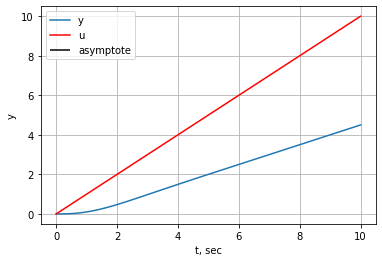# Inverse Laplace Transform¶

• Pole/Residue Decomposition
• Partial fraction decomposition
• Final value theorem

## Pole/Residue Decomposition¶

In :
%matplotlib inline
import control
import sympy
import scipy.signal as sig
import numpy as np
import matplotlib.pyplot as  plt

In :
s = control.tf([1, 0], [0, 1])
G = (s**2 + 1)/(s**2 + 2*s + 3)
G

Out:
$$\frac{s^2 + 1}{s^2 + 2 s + 3}$$
In :
r_vect, p_vect, k = sig.residue(G.num, G.den)
r_vect, p_vect, k

Out:
(array([-1.+0.j, -1.+0.j]),
array([-1.+1.41421356j, -1.-1.41421356j]),
array([1.]))

We now reconstruct the signal using the inverse laplace transform for each pole/residue term.

$g(t) = \mathcal{L}^{-1}\left[\sum\limits_{i=1}^{k} \dfrac{R_i}{s-p_i}\right] = \sum\limits_{i=1}^{k} R_i e^{p_it}$

The residue may be found in general even for for repeated roots or order $m$, using:

$F(s) = \dots + \sum\limits_{k=0}^{m-1}\dfrac{r_k}{(s-p)^{m-k}}$

$r_k = \dfrac{1}{k!}\left[ \dfrac{d^k}{ds^k} (s-p)^m F(s)\right]_{s=p}$

In :
t = np.linspace(0, 20, 100)
y = np.zeros(len(t), dtype=np.complex)
for r, p in zip(r_vect, p_vect):
y += r*np.exp(p*t)
assert np.linalg.norm(np.imag(y) < 1e-10)  # make sure imaginary is negligible
y = np.real(y)


We compare this to the impulse response of $G(s)$, which should be the same.

In :
t_imp, y_imp = control.impulse_response(G, T=t)
plt.plot(t_imp, y_imp, 'k', linewidth=5, label='impulse response')
plt.plot(t, y, 'y', linewidth=3, label='inverse laplace')
plt.grid()
plt.legend()

/home/jgoppert/anaconda3/envs/aae301/lib/python3.7/site-packages/control/timeresp.py:703: UserWarning: System has direct feedthrough: D != 0. The infinite impulse at t=0 does not appear in the output.
Results may be meaningless!
warnings.warn('System has direct feedthrough: D != 0. The infinite '

Out:
<matplotlib.legend.Legend at 0x7f38f2599518>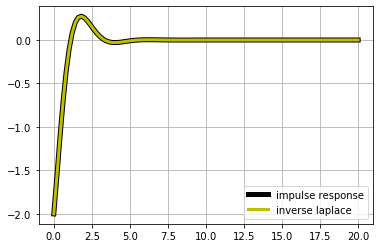## DC Motor Example¶The torque, $T$, is proportional to the current, $i$ with constant $K$:

$T = K i$

The back EMF is proportional to the shaft rotational speed with constant $K$:

$e = K\dot{\theta}$

The equation of motion for the motor shaft is:

$J \ddot{\theta} + b \dot{\theta} = T$

Kirchhoff's voltage law gives:

$L \dfrac{di}{dt} + Ri = V - e$

Equations of motion and transfer function derived in hotseat exercise.

## Final Value Theorem¶

In :
G2 = 1/ (s*(s**2 + 2*s + 2))
G2

Out:
$$\frac{1}{s^3 + 2 s^2 + 2 s}$$

The final value theorem, is typicaly stated as:

If $s Y(s)$ has poles with only negative real part, $s Y(s)$ is stable, then:

$\lim\limits_{t \rightarrow \infty} y(t) = \lim\limits_{s \rightarrow 0} s Y(s)$

Note that this definition is a more general than the version in the notes which may be considered to be a DC gain theorem and only applies for constant input signals. The version stated here applies for arbitary input signals, $u(t)$, where the output $y(t)$, has a Laplace transform given by $Y(s) = G(s)U(s)$.

Stated another way:

$\lim\limits_{t \rightarrow \infty} y(t) = \lim\limits_{s \rightarrow 0} s G(s) U(s)$

If we assume u is a constant, u_0:

$\lim\limits_{t \rightarrow \infty} y(t) = \lim\limits_{s \rightarrow 0} s G(s) (u_0/s) = \lim\limits_{s \rightarrow 0} G(s) u_0 = G(0) u_0$

And we then arrive with the theorem given in the notes:

$\lim\limits_{t \rightarrow \infty} y(t) = G(0) u_0$

In :
def do_analysis(G, U_list):
for U in U_list:
Y = control.minreal(G*U)
final_value = np.real(control.minreal(s*Y).horner(0))

print('U:', U)
print('final value:', final_value)

plt.figure()
t, u = control.impulse_response(U, T=np.linspace(0, 10))
t, y = control.impulse_response(G*U, T=np.linspace(0, 10))
plt.hlines(final_value, t, t[-1], label='asymptote')
plt.plot(t, y, label='y')
plt.plot(t, u, 'r', label='u')
plt.xlabel('t, sec')
plt.ylabel('y')
plt.legend()
plt.grid()
plt.show()

In :
do_analysis(G2, [1, 2, 1/s, 1/s**2])

0 states have been removed from the model
1 states have been removed from the model
U: 1
final value: 0.5000000000000002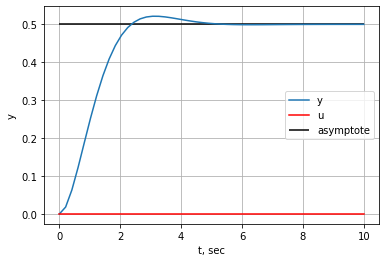0 states have been removed from the model
1 states have been removed from the model
U: 2
final value: 1.0000000000000004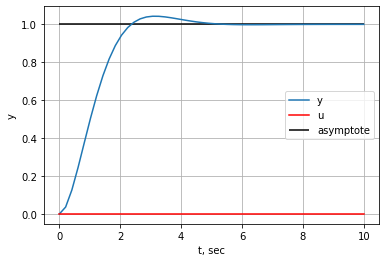0 states have been removed from the model
1 states have been removed from the model
U:
1
-
s

final value: inf

/home/jgoppert/anaconda3/envs/aae301/lib/python3.7/site-packages/control/xferfcn.py:610: RuntimeWarning: divide by zero encountered in double_scalars
polyval(self.den[i][j], s))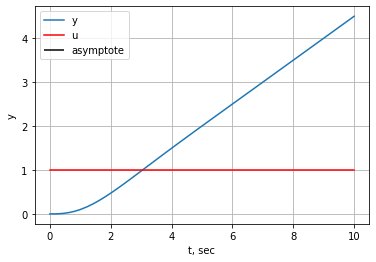0 states have been removed from the model
1 states have been removed from the model
U:
1
---
s^2

final value: inf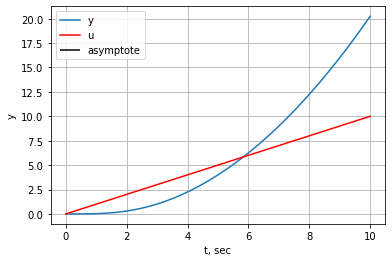In :
do_analysis(G2*s, [1, 2, 1/s, 1/s**2])

1 states have been removed from the model
0 states have been removed from the model
U: 1
final value: 0.0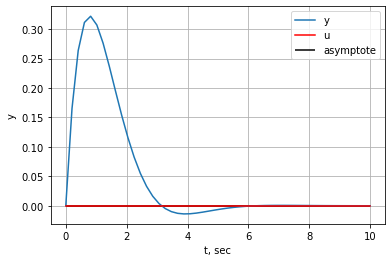1 states have been removed from the model
0 states have been removed from the model
U: 2
final value: 0.0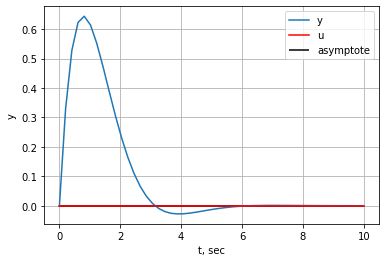1 states have been removed from the model
1 states have been removed from the model
U:
1
-
s

final value: 0.5000000000000002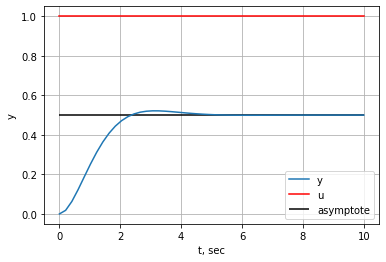1 states have been removed from the model
1 states have been removed from the model
U:
1
---
s^2

final value: inf